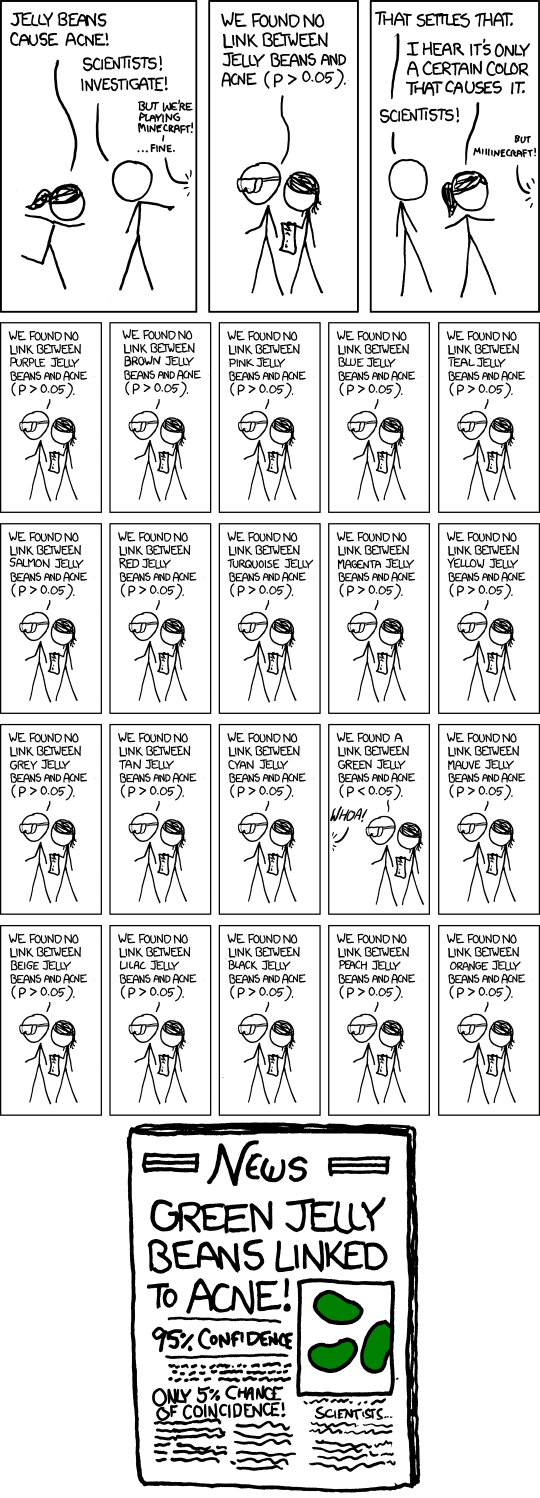# Digest: Why Most Published Research Findings Are False

Published on

## Preface

This article aims to provide some of the derivations from Ioannidis' 2005 article: "Why Most Published Research Findings Are False" which exposed what has since been termed "The Replication Crisis."

The issue begins with the subject of $p$-values which measure the probability of a study finding a positive result, assuming the presented hypothesis is false. A strong $p$-values is considered to be $0.05$, indicating a regrettable 5% of published findings are false.

Before diving into the derivations, some examples:

## Example 1

Suppose we represent all possible hypotheses that can be tested with a more manageable 100,000 hypotheses. Let's allow a generous 50:50 true:false split for this set as well as a statistical power of 80%.

TrueFalseTotal
positive result $+$40k2.5k42.5k
negative result $-$10k47.5k57.5k
Total50k50k100k

Here, the $p$-value $= \alpha = P(+ \vert \text{ f })$ where $+$ is a positive relationship, and $\text{f}$ is a flase result. The statistical power $= P(+ \vert \text{ t })$ and Positive Predictive Value $\text{PPV} = \frac{\text{statistical power}}{P(\text{ t } + \text{ f } \vert +)} = \frac{40k}{42.5k} \approx 0.94$ which is pretty satisfactory given our generous values.

## Example 2

Once again, we'll take 100,000 hypotheses, but now with a 10:90 true:false split for this set as well as a statistical power of 80%. Filling out the table we get:

TrueFalseTotal
$+$8k4.5k12.5k
$-$2k85.5k87.5k
Total10k90k100k

Here, $\text{PPV} = \frac{8k}{12.5k} = 0.64$ which is significantly worse than the assumed 95% if the study is positive without publicaiton bias, cheating etc. which is covered below.

Before getting much further, it will be useful to define a glossary

SymbolValueMeaning
$p$$P(+ \vert \text{ f })$probability of a study finding a positive result, given that the hypothesis is false
$\text{PPV}$$\frac{\text{statistical power}}{P(\text{ t } + \text{ f } \vert +)}$Positive Predictive Value
$R$$\frac{P(\text{ t } )}{P(\text{ f } )}$the pre-study odds of the hypothesis is tested
$\varTheta$$= R = \frac{P}{1 - P}$an alternate expression of probability, e.g. 10:90 odds: $\varTheta = \frac{10\%}{100\% - 10\%}$
$P(\text{f})$$1 - P(\text{ t })$compliment rule
$\alpha$$P( + \vert \text{ f })$Type I Error
$\beta$$P( - \vert \text{ t })$Type II Error
$P(\text{t } \vert +)$$\frac{P(\text{t}) \cdot P(+ \vert \text{ t })}{P(t)}$Bayes Rule
$P(\text{t} \land +)$$P(\text{ t } \vert +) \cdot P(\text{t})$Product Rule
$u$bias factor influenced by $p$-hacking, conflict of interest, competitive publication motivations, etc.

## Table 1

Now we can recreate the general table for all such examples above and derive their values:

TrueFalseTotal
$+$$\frac{c(1 - \beta)R}{R+1}$$\frac{c \alpha}{R+1}$$\frac{c(R+\alpha-\beta R)}{R+1}$
$-$$\frac{c \beta R}{R+1}$$\frac{c(1-\alpha)}{R+1}$$\frac{c(1-\alpha + \beta R)}{R+1}$
Total$\frac{cR}{R+1}$$\frac{c}{R+1}$$c$ the number of relationships tested

## Derivations

Starting with the top left cell which represents:

$P(+ \land \text{ t }) = \underbrace{P(+ \vert \text{ t })}_{\text{\S}} \cdot \underbrace{P(\text{t})}_{\frac{R}{R+1}}$

$\quad \text{\S} 1.1: \beta = P(- \vert \text{ t })$

$\quad \text{\S} 1.2: P(+ \vert \text{ t }) + P(- \vert \text{ t }) = 1$

$\quad \text{\S} 1.3: P(+ \vert \text{ t }) + P(- \vert \text{ t }) = 1$

$\quad \text{\S} \therefore P(+ \vert \text{ t }) = 1 - \beta$

$= (1- \beta )(\frac{R}{R+1})= \frac{c(1 - \beta )R}{R+1}$

Similarly, for the top-middle cell:

$P(+ \land \text{ f }) = \underbrace{P(+ \vert \text{ f })}_{\alpha} \cdot \underbrace{P(\text{f})}_{1 - \frac{R}{R+1}}$

$= \alpha (1 - \frac{R}{R+1}) = \frac{c \alpha}{R+1}$

So, for all true positives, the top-right cell:

$\frac{\text{true positives}}{{\text{all positives}}} = \frac{\frac{c(1- \beta )R}{R+1}}{\frac{c(R+ \alpha - \beta R)}{R+1}} = \frac{(1 - \beta ) R}{ R + \alpha - \beta R} = \underbrace{P(\text{ t } \vert +)}_{\text {want this bad boi to be high}}$ in terms of Type I, II error and pre-study odds.

## When is a Study More Likely to be True than False?

$P(\text{ t } \vert +) > \frac{1}{2}$

$\rArr \frac{(1 - \beta ) R}{ R + \alpha - \beta R} > \frac{1}{2}$

$\rArr 2(\frac{(1 - \beta ) R}{ R + \alpha - \beta R}) > 2( \frac{1}{2})$

$\rArr 2(1 - \beta )R > R + \alpha - \beta R$

$\rArr R(1 - \beta ) > \alpha \iff (\text{pre-study odds})(\text{statistical power}) > p\text{-value}$

Some fields of study have inherently small $R$ or $(1 - \beta)$ values

## What Happens if we Introduce Bias?

$P(+ \land \text{ f }) = P(+ \vert \text{ f }) \cdot P(\text{f}) \underset{bias}{\longrightarrow}$ negative study results become positive with $P(u)$

This can alter our outcome by in two cases:

$\text{1. } P(\underbrace{+}_{\text{for any reason}} \land \text{t})$

$= \underbrace{P(+ \vert \text{ t })}_{1 - \beta} \cdot \underbrace{P(\text{t})}_{\frac{R}{R+1}} + u \cdot \underbrace{P(- \vert \text{ t })}_{\text{Type II Error: } \beta} \cdot P(\text{t})$

$= (1 - \beta )(\frac{R}{R+1}) + u \beta \frac{R}{R+1}$

$= \frac{1 - \beta R + u \beta R}{R+1}$

$\text{2. } P(\underbrace{+}_{\text{for any reason}} \land \text{f})$

$= P(+ \vert \text{ f }) \cdot \underbrace{P(\text{f})}\_{1-P(t)} + u \cdot P(- \vert \text{ f }) \cdot P(\text{f})$

$= \alpha (1-\frac{R}{R+1}) + u(1 - \alpha)(1-\frac{R}{R+1})$ Note that these truths/falsehoods have to be independent of the decision making otherwise they would impair judgement, disallowing us from applying the Product Rule

$= \frac{\alpha + u(1 - \alpha)}{R+1}$

## The Issue of Incorrect pre-publication $p$-values.

Research efforts do not occur in isolation. Several teams may be independently, competitive working on the same hypotheses over and over and over again without adjusting their $p$-values.

Relevant xkcd:This means that statistical power decreases as the experiments are repeated:

$P(\underbrace{+_{\tiny > 0}}_{\text{with n studies}} \land \text{ t })$

$= P(\underbrace{+}\_{\text{\S}} \land \text{ t }) \cdot P(\text{t})$

$\quad \text{\S}2.1: P(+ \land \text{ t }) = \displaystyle\sum^{n} P_n$ which could be ... a lot of probabilities

$\quad \text{\S}2.2: (1 - P(-_{\forall \tiny n} \vert \text{ t })) \cdot P(\text{t})$ which is the negative results of all $n$ studies

$\quad \text{\S}2.3: (1 - \beta^n) \cdot P(\text{t})$

$= \frac{R(1 - \beta^n)}{R+1}$

Meaning that for each subsequent, competing trial, the likelihood of your own $p$-value genuinely being sufficiently small decreases.